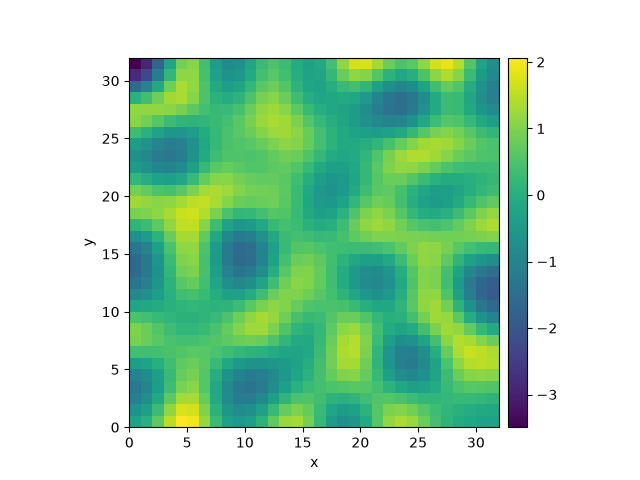# 2.18. Kuramoto-Sivashinsky - Using custom class¶

This example implements a scalar PDE using a custom class. We here consider the Kuramoto–Sivashinsky equation, which for instance describes the dynamics of flame fronts:

$\partial_t u = -\frac12 |\nabla u|^2 - \nabla^2 u - \nabla^4 u$Out:

  0%|          | 0/10.0 [00:00<?, ?it/s]
Initializing:   0%|          | 0/10.0 [00:00<?, ?it/s]
0%|          | 0/10.0 [00:00<?, ?it/s]
2%|2         | 0.25/10.0 [00:06<04:06, 25.29s/it]
3%|3         | 0.34/10.0 [00:06<02:59, 18.61s/it]
16%|#6        | 1.64/10.0 [00:06<00:32,  3.89s/it]
70%|#######   | 7.03/10.0 [00:06<00:02,  1.06it/s]
70%|#######   | 7.03/10.0 [00:06<00:02,  1.04it/s]
100%|##########| 10.0/10.0 [00:06<00:00,  1.48it/s]
100%|##########| 10.0/10.0 [00:06<00:00,  1.48it/s]


from pde import PDEBase, ScalarField, UnitGrid

class KuramotoSivashinskyPDE(PDEBase):
"""Implementation of the normalized Kuramoto–Sivashinsky equation"""

def evolution_rate(self, state, t=0):
"""implement the python version of the evolution equation"""
state_lap = state.laplace(bc="auto_periodic_neumann")
state_lap2 = state_lap.laplace(bc="auto_periodic_neumann")
return -state_grad.to_scalar("squared_sum") / 2 - state_lap - state_lap2

grid = UnitGrid([32, 32])  # generate grid
state = ScalarField.random_uniform(grid)  # generate initial condition

eq = KuramotoSivashinskyPDE()  # define the pde
result = eq.solve(state, t_range=10, dt=0.01)
result.plot()


Total running time of the script: ( 0 minutes 6.876 seconds)

Gallery generated by Sphinx-Gallery